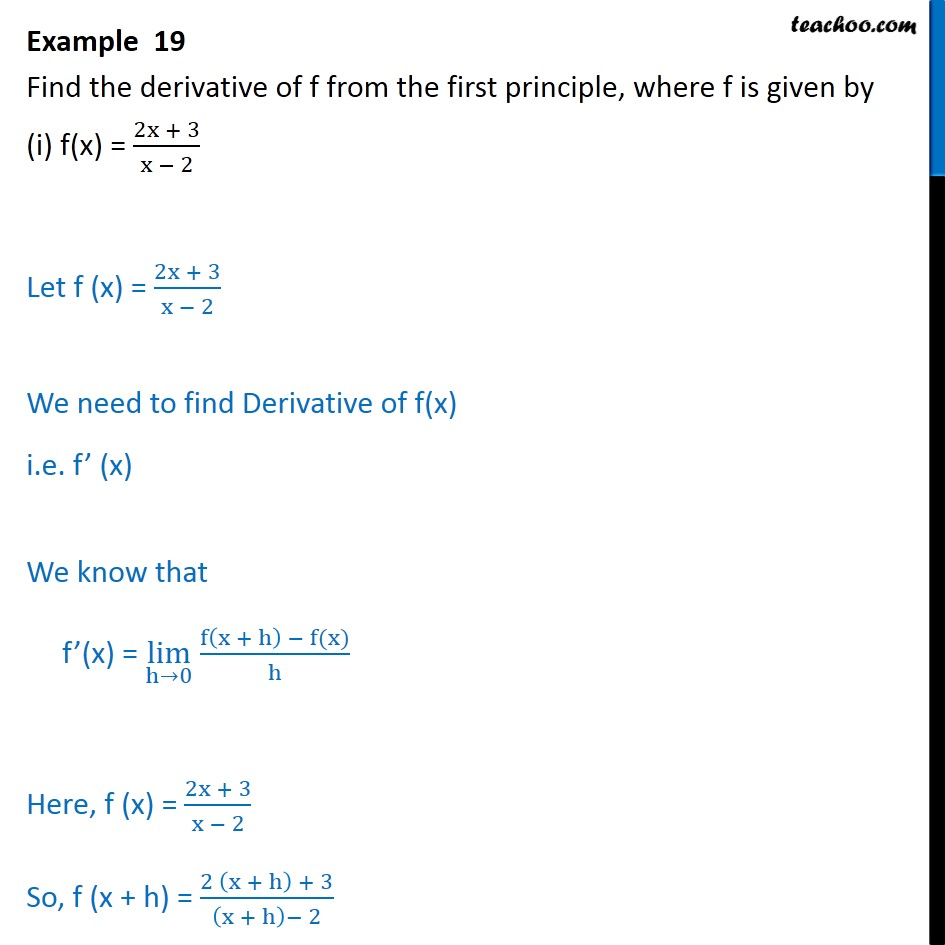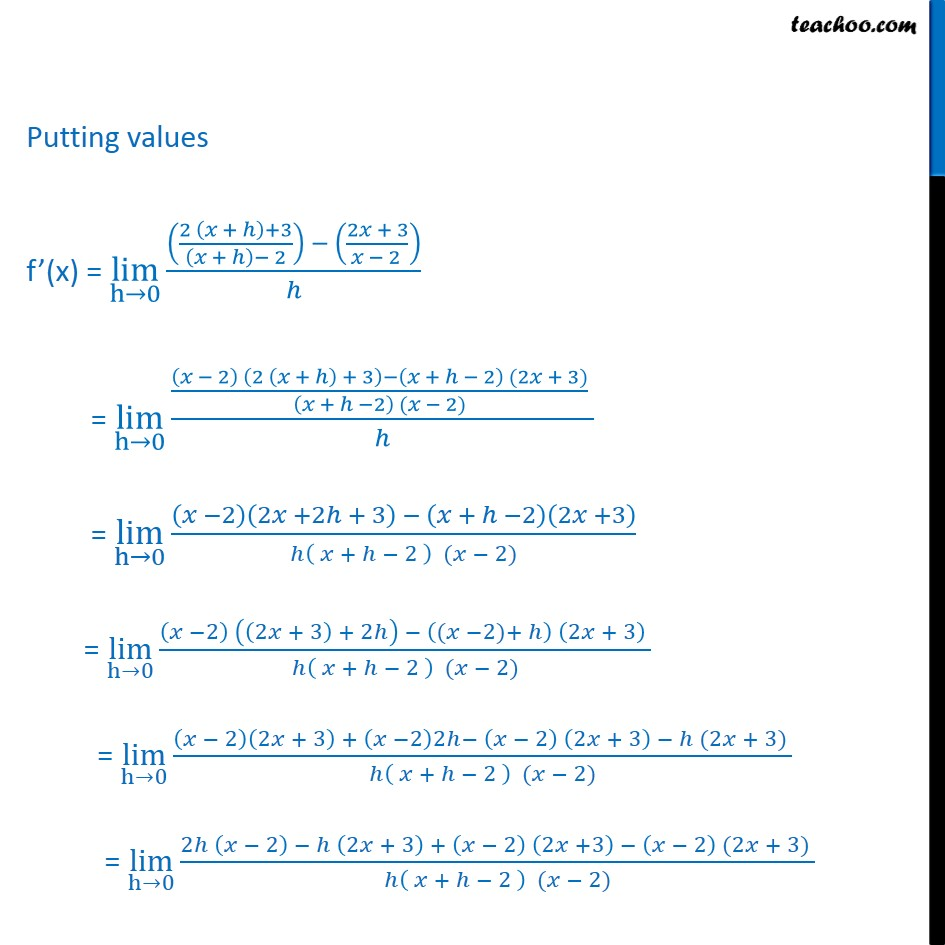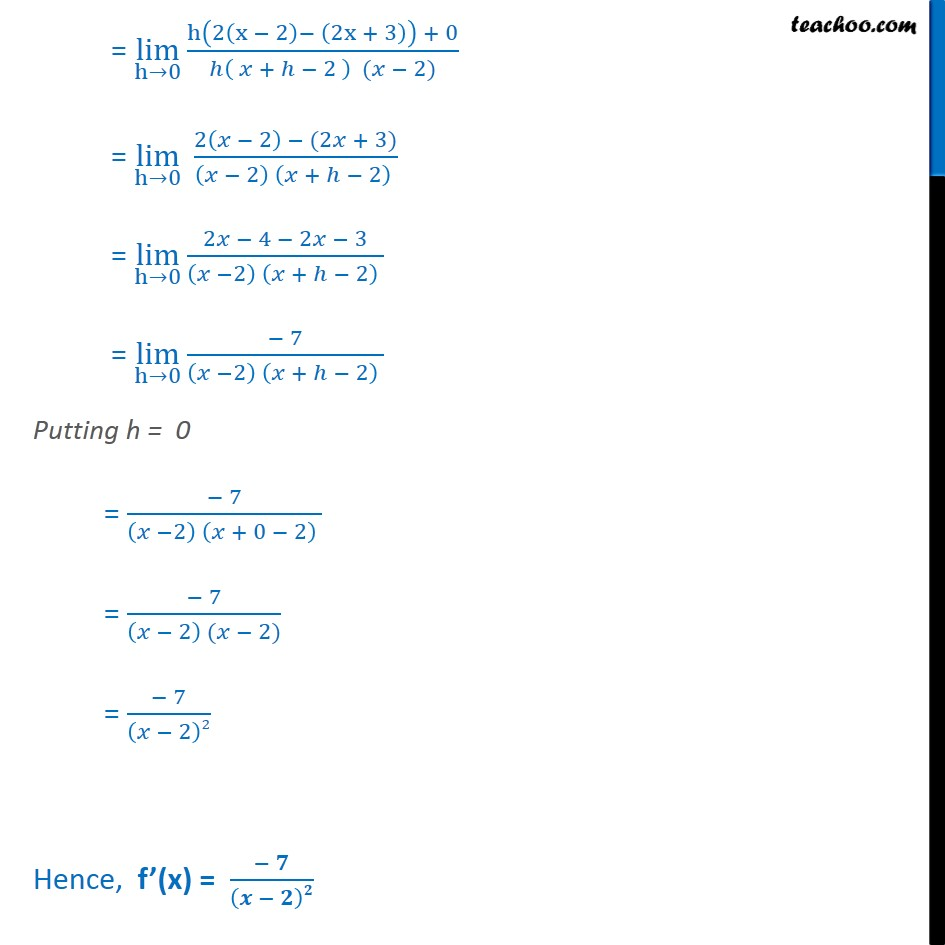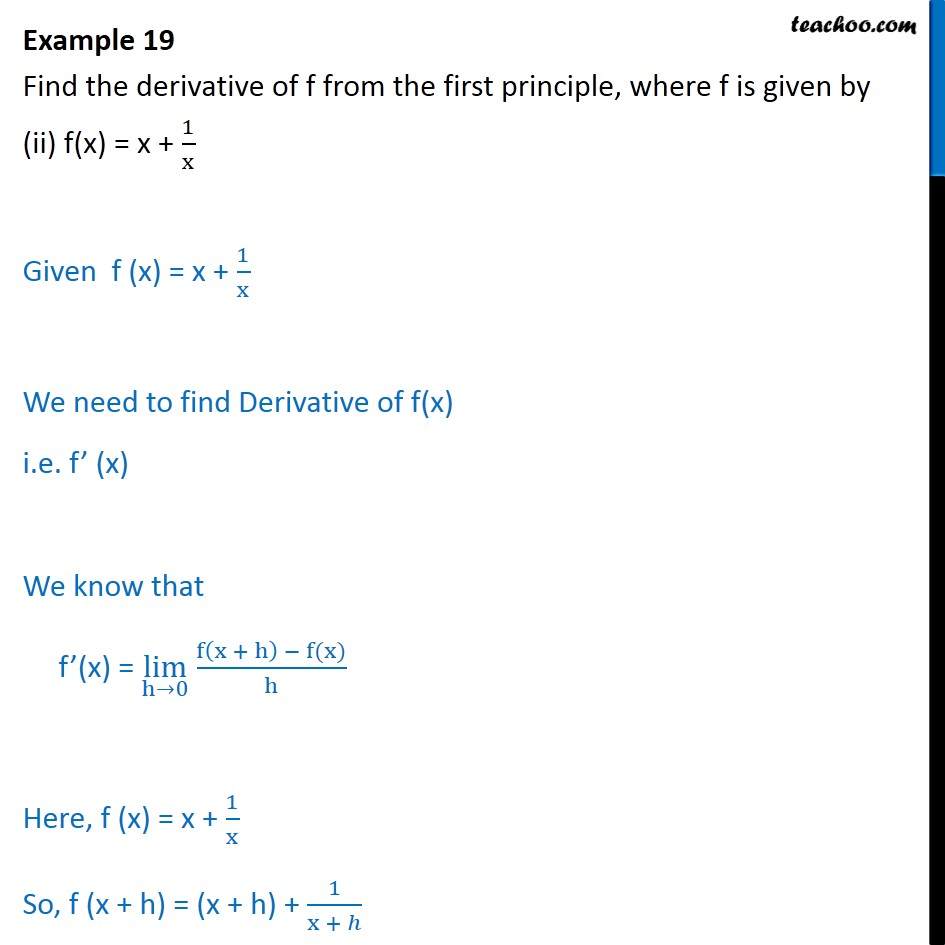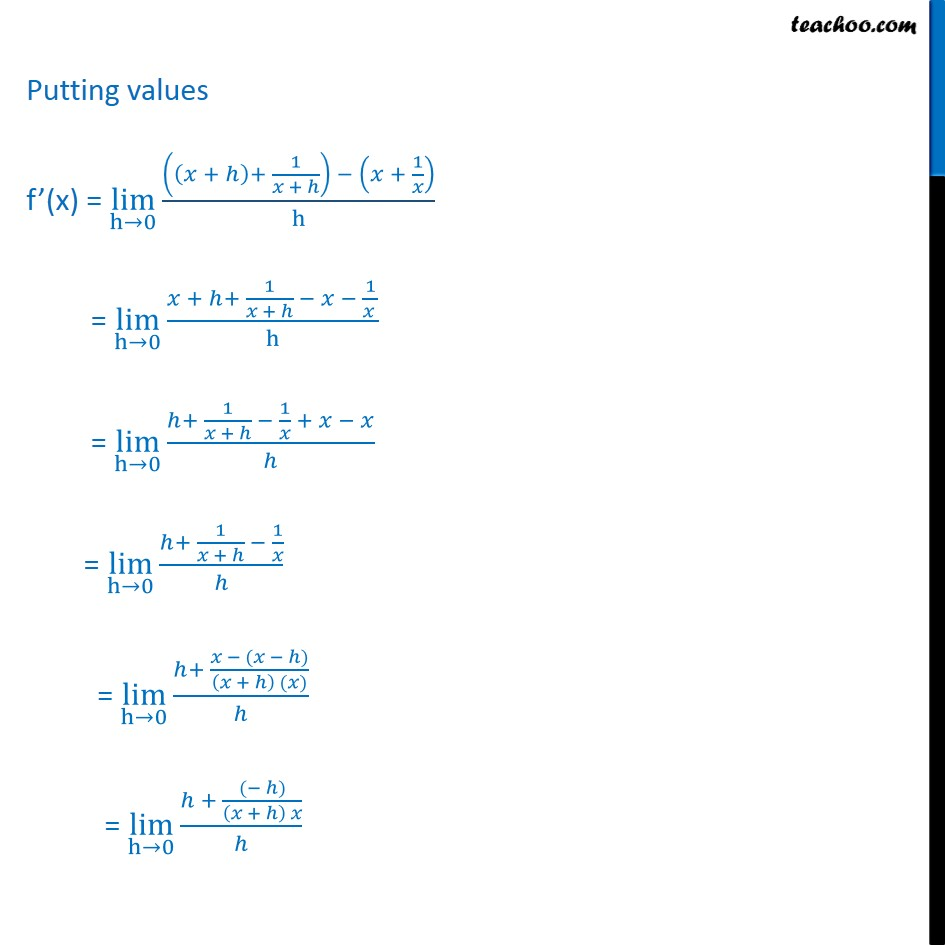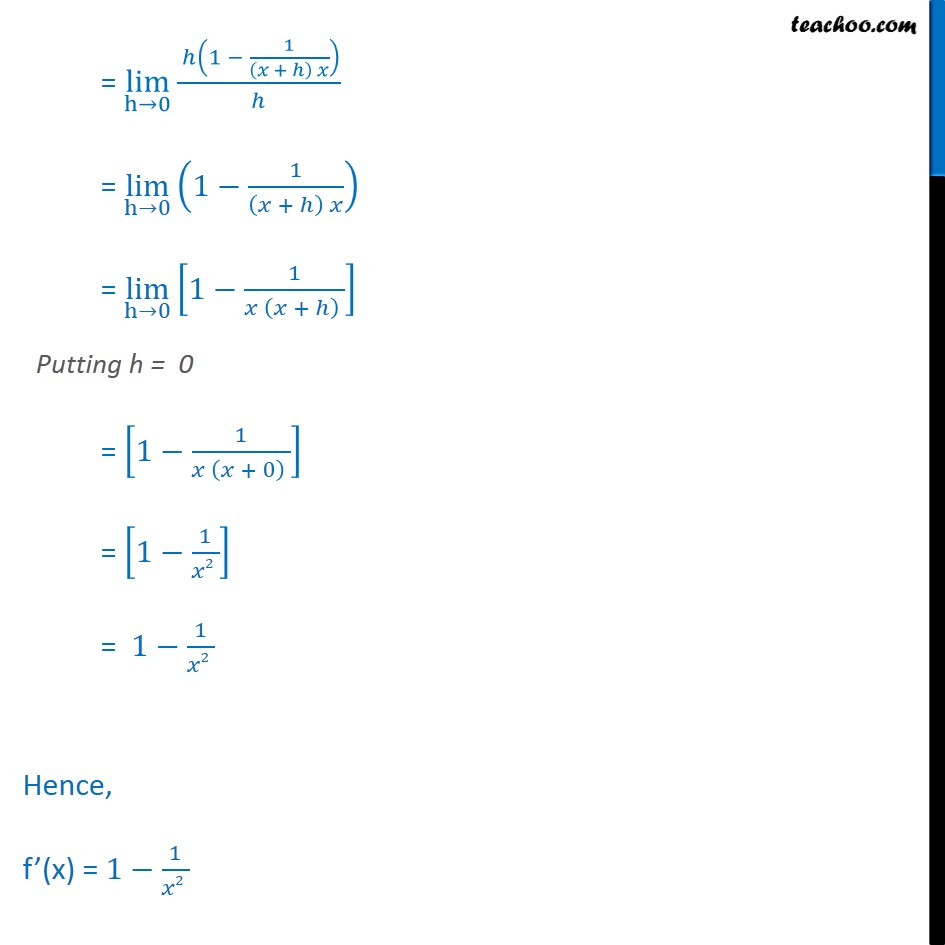1. Chapter 13 Class 11 Limits and Derivatives
2. Serial order wise
3. Examples

Transcript

Example 19 Find the derivative of f from the first principle, where f is given by (i) f(x) = 2x + 3 x 2 Let f (x) = 2x + 3 x 2 We need to find Derivative of f(x) i.e. f (x) We know that f (x) = lim h 0 f x + h f(x) h Here, f (x) = 2x + 3 x 2 So, f (x + h) = 2 x + h + 3 x + h 2 Putting values f (x) = lim h 0 2 + +3 + 2 2 + 3 2 = lim h 0 2 2 + + 3 + 2 (2 + 3) + 2 ( 2) = lim h 0 2 2 +2 + 3 + 2 2 +3 + 2 ( 2) = lim h 0 2 2 + 3 + 2 ( 2)+ 2 + 3 + 2 ( 2) = lim h 0 2 2 + 3 + 2 2 2 2 + 3 (2 + 3) + 2 ( 2) = lim h 0 2 2 2 + 3 + 2 2 +3 2 (2 + 3) + 2 ( 2) = lim h 0 h 2 x 2 2x + 3 + 0 + 2 ( 2) = lim h 0 2 2 (2 + 3) 2 + 2 = lim h 0 2 4 2 3 2 + 2 = lim h 0 7 2 + 2 Putting h = 0 = 7 2 + 0 2 = 7 2 ( 2) = 7 2 2 Hence, f (x) = Example 19 Find the derivative of f from the first principle, where f is given by (ii) f(x) = x + 1 x Given f (x) = x + 1 x We need to find Derivative of f(x) i.e. f (x) We know that f (x) = lim h 0 f x + h f(x) h Here, f (x) = x + 1 x So, f (x + h) = (x + h) + 1 x + Putting values f (x) = lim h 0 + + 1 + + 1 h = lim h 0 + + 1 + 1 h = lim h 0 + 1 + 1 + = lim h 0 + 1 + 1 = lim h 0 + ( ) + ( ) = lim h 0 + ( ) + = lim h 0 1 1 + = lim h 0 1 1 + = lim h 0 1 1 + Putting h = 0 = 1 1 + 0 = 1 1 2 = 1 1 2 Hence, f (x) = 1 1 2

Examples